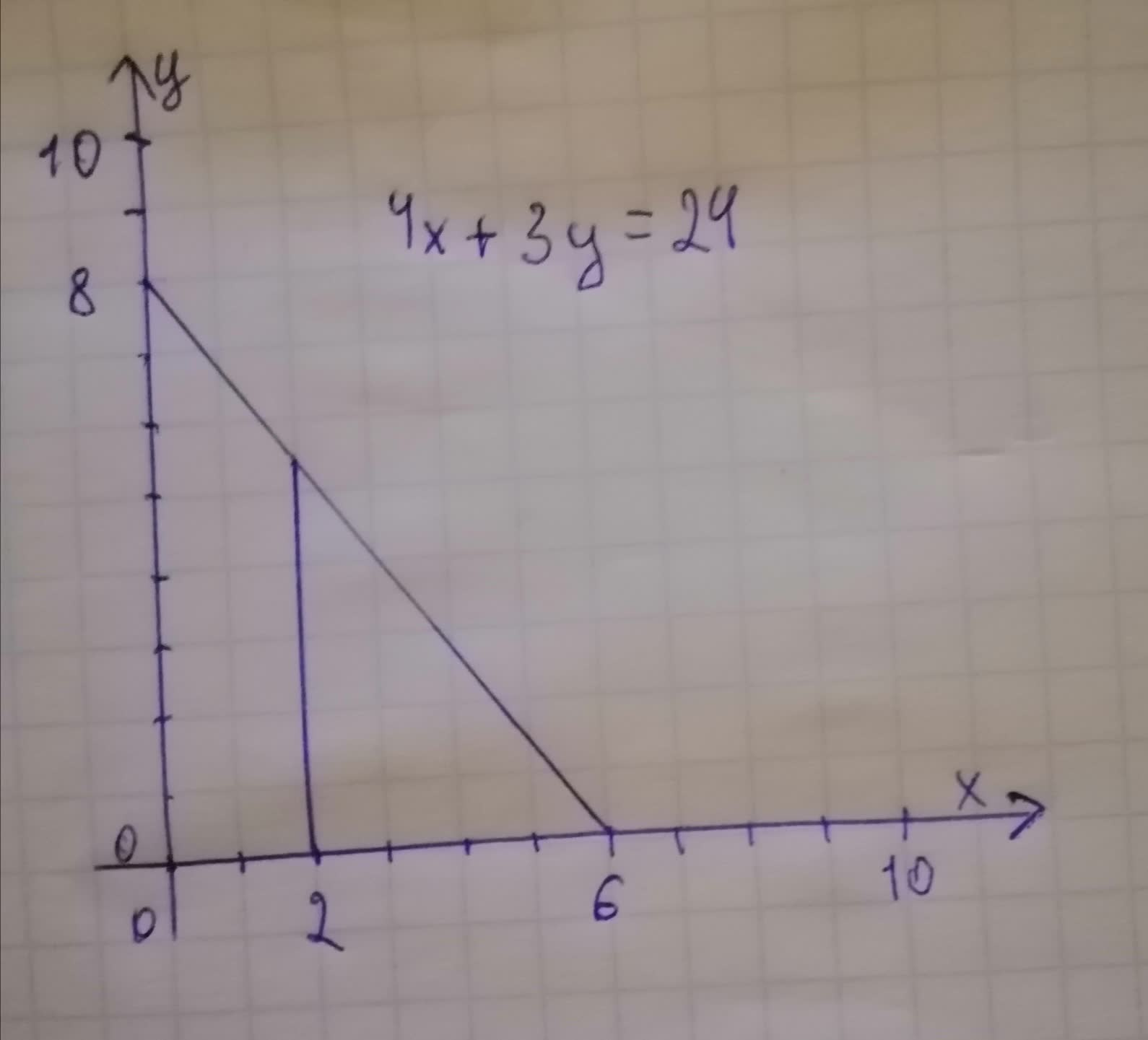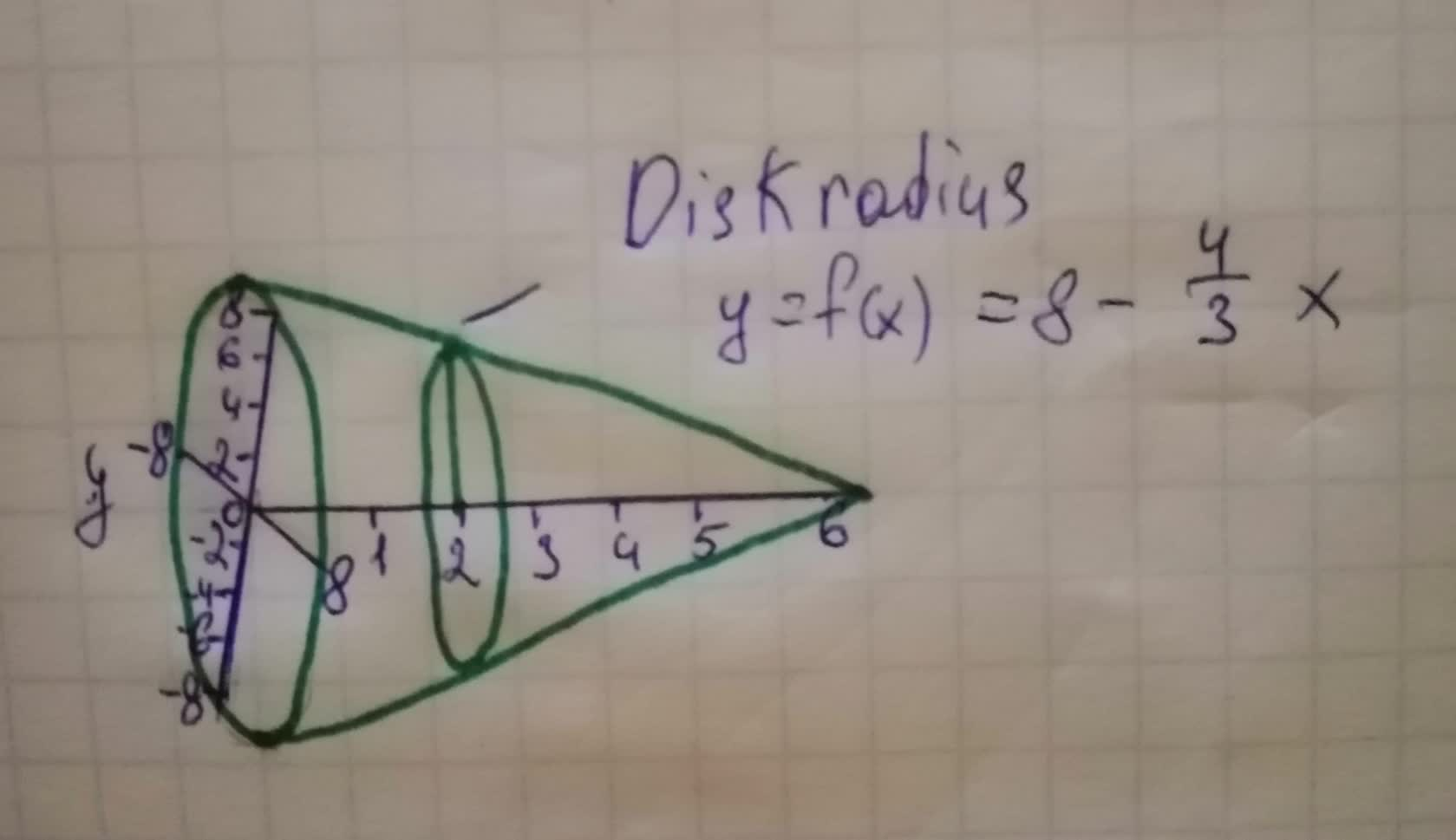# Find the volume of the solid generated by revolving the standed region about thetotalmente80sm9 2021-11-21 Answered
Find the volume of the solid generated by revolving the standed region about the x-axis.Te volume of the solid is ? cubic units.
The equation: $4x+3y=24$
You can still ask an expert for help

• Questions are typically answered in as fast as 30 minutes

Solve your problem for the price of one coffee

• Math expert for every subject
• Pay only if we can solve itPhilip O'Neill
Step 1
$⇒y=8-\left(\frac{4}{3}\right)x$
$⇒y=4×\left(2-\left(\frac{1}{3}\right)x\right)$
for x intercept, $y=0$
$0=4×\left(2-\left(\frac{1}{3}\right)x\right)$
$\left(\frac{1}{3}\right)x=2$
$x=6$
Volume generated by rotating the given region about x axis by washer method $v={\int }_{0}^{6}\pi {\left[4×\left(2-\left(\frac{1}{3}\right)x\right)\right]}^{2}dx$
$v={\int }_{0}^{6}16\pi \left[{2}^{2}-2×2\left(\frac{1}{3}\right)x+{\left(\left(\frac{1}{3}\right)x\right)}^{2}\right]dx$
$v={\int }_{0}^{6}16\pi \left[4-\left(\frac{4}{3}\right)x+\left(\frac{1}{9}\right){x}^{2}\right]dx$
$v={\int }_{0}^{6}16\pi \left[4x-\left(\frac{4}{3}\right)\left(\frac{1}{2}\right){x}^{2}+\left(\frac{1}{9}\right)\left(\frac{1}{3}\right){x}^{3}\right]$
$v={\int }_{0}^{6}16\pi \left[4x-\left(\frac{2}{3}\right){x}^{2}+\left(\frac{1}{27}\right){x}^{3}\right]$
$v=16\pi \left[4×6-\left(\frac{2}{3}\right){6}^{2}+\left(\frac{1}{27}\right){6}^{3}\right]-16\pi \left[4×0-\left(\frac{2}{3}\right){0}^{2}+\left(\frac{1}{27}\right){0}^{3}\right]$
$v=16\pi \left[4×6-\left(2×\frac{36}{3}\right)+\left(\frac{216}{27}\right)\right]$
$v=16\pi \left[24-24+8\right]$
$v=128\pi$
Volume $=128\pi =402$
###### Not exactly what you’re looking for?Witheyesse47

Step 1
The disk formed when the skethched region revolves around the x-axis is shown below:The given equation is, $4x+3y=24$
$3y=24-4x$
$y=8-\frac{4}{3}x$
To get x-intercept, equate $y=0$
$y=8-\frac{4}{3}x=0$
$8=\frac{4}{3}x$
$x=6$
Here, the disk radius $y=f\left(x\right)=8-\frac{4}{3}x$
And also x varies from 0 to 6.
Step 2
Volume of the solid of revolution obtained the given region about the x-axis is,
$V=\pi {\int }_{a}^{b}{\left[f\left(x\right)\right]}^{2}dx$
$=\pi {\int }_{0}^{6}{\left[8-\frac{4}{3}x\right]}^{2}dx$
$=\pi {\int }_{0}^{6}\left[64+\frac{16}{9}{x}^{2}-\frac{64}{3}x\right]dx$
$=\pi {\left[64x+\frac{64}{9}\left(\frac{{x}^{3}}{3}\right)-\frac{64}{3}\left(\frac{{x}^{2}}{2}\right)\right]}_{0}^{6}$
$=\pi \left[384+128-384\right]$
$=128\pi$
$=402.12$
Therefore the required volume of the solid is,
$=128\pi$ cubic units
$=402.12$ cubic units